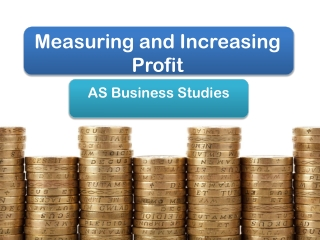DownloadDownload PresentationMeasuring and Increasing Profit

# Measuring and Increasing Profit

Télécharger la présentation## Measuring and Increasing Profit

- - - - - - - - - - - - - - - - - - - - - - - - - - - E N D - - - - - - - - - - - - - - - - - - - - - - - - - - -
##### Presentation Transcript

1. Measuring and Increasing Profit AS Business Studies

2. Aims & Objectives Aim: • Understand ways of measuring profit. Objectives: • Define gross profit, net profit & ROCE • Calculate gross profit, net profit & ROCE • Analyse gross profit, net profit & ROCE

3. Starter • What is the formula for calculating profit? • How can profit be improved?

4. Profit • Profit = Total Revenue – Total Costs Methods of Increasing Profits • Increasing no. of units sold with same price and cost per unit • Increase profit made on each unit by increasing selling price per unit or reducing cost per unit

5. Gross Profit & Net Profit • Gross Profit = Sales Revenue – Variable Costs • Net Profit = Sales Revenue – Total Costs (FC+VC) • If gross profit increases and there is no increase in fixed costs then net profit will increase too!

6. Profit Margins • The profit made as a proportion of sales revenue • Sales = £100 • Profit =£30 • Profit Margin is 30% Sales £100 Profit £30

7. Gross Profit Margin • Shows percentage of sales that is gross profit. • Gross Profit Margin (%) = (Gross Profit / Sales) x100 • Eg. (£150,000/£250,000) x 100 = 67% • For every £1 of sales 67p is gross profit.

8. Net Profit Margin • Shows percentage of sales that is net profit • Net Profit Margin (%) = (Net Profit / Sales) x100 • Eg. (£70,000/£250,000) x 100 = 28% • For every £1 of sales 23p is net profit.

9. Worksheet Question 1) • A T-shirt retailer, T-Design, sells 50,000 units in 1 year at £5 each. Each T-shirt is purchased by the shop for £2.00 each. Annual fixed costs are £80,000. Calculate: • Gross Profit • Net Profit

10. Worksheet Question 2) • The following year the shop again sells 50,000 units for £5 each but gross profit increases to £160,000 and net profit to £90,000. Calculate the new: • Gross Profit Margin • Net Profit Margin

11. Measuring Profitability - ROCE • Compare profit made with the value of capital invested – Return on Capital Employed • ROCE (%) = (Net Profit/Capital Invested) x 100 • E.g. (4,000/12,000) x 100 = 33.3% • For every £1 invested, 33.3p is net profit

12. ROCE • The higher the percentage the more profitable the investment. • Indicates the efficiency of the management in managing the investment. • Can be compared between projects or businesses. • ROCE can be increased by: • Increasing profit without investing any more capital • Make the same level of profit but with less capital expenditure

13. Worksheet Question 1) • Winston is an entrepreneur looking to start up a sports shop. Winston plans to invest £12,000 in the new business. He has budgeted net profit of £3,000 for the first year of operation. Calculate: • The expected return on capital. • What does this mean? • Why should Winston be worried if another similar business is making a return on capital of 20% each year?

14. Worksheet In year 2 Winston plans to invest a further £11,400 in the sports shop. He has budgeted profit of £3,800 for the second year of operation. Calculate: • Return on capital employed for year 2 • How does this ROCE figure compare to year 1? • What factors may have contributed to the change in the ROCE figure?

15. Plenary Give the equations for the following: • Gross Profit • Net Profit • ROCE